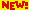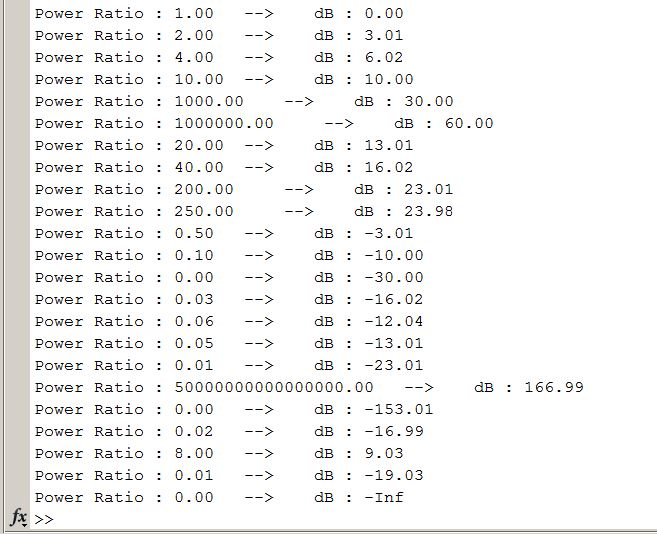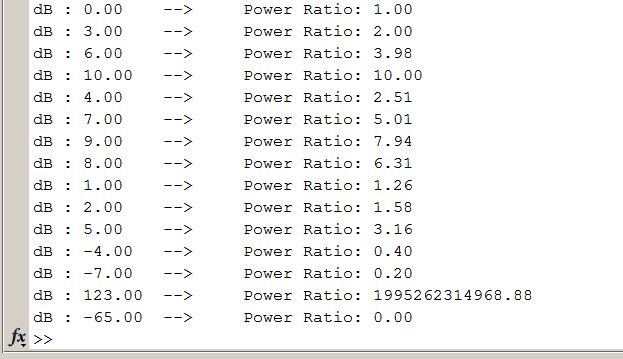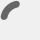###### Entrepreneur, Tech CTO, Software Developer, Electronics Engineer, Author

Question 2 solutionsQuestion 1 solutions

`Workshop Examples Sheet A`
• Question 1:
```% Calculating dB from given power ratios
% Written by Ibrahim Ozturk

% Question 1
% Given power ratios : 1, 2, 4, 10, 1000, 1000000, 20,
% 40, 200, 250, 1/2, 1/10, 1/1000,
% 0.025, 1/16, 1/20, 1/200, 5*10^16,5*10^-16,
% 2/100, 8, 1/80, 0

power_ratios = [1, 2, 4, 10, 1000, 1000000, 20, ...
40, 200, 250, 1/2, 1/10, 1/1000, ...
0.025, 1/16, 1/20, 1/200, 5*10^16,5*10^-16, ...
2/100, 8, 1/80, 0];

%Convert power ratio to db
% You can use this MATLAB function :
% ydb = pow2db(y) --> Convert power to decibels (dB)
% Or you can use your own function as :
% ydb = 10*1og10(y) --> Convert power to decibels (dB)

%Results of Question 1

%Conversion
dbouts = 10*log10(power_ratios);

%Display results
for i = 1 : length(power_ratios)
fprintf('Power Ratio : %.2f\t --> \tdB : %.2f \n',power_ratios(i),dbouts(i));
end
```

Results :• Question 2:
```%%%%%%%%%%%%%%%%%%%%%%%%%%%%%%%%%%%%%%%%%%%%%%%%%%%%%%%%%%%%%%
% Question 2
% Given dBs : 0     3   6   10  4   7    9      8   1   2
%             5     -4  -7  123 -65

dbs = [0   3   6   10  4   7    9      8   1   2  5  -4  -7  123 -65];

%Convert db to power ratio
%   You can use this MATLAB function :
%      y = db2pow(ydb) --> Convert decibels (dB) to power
%   Or you can use your own function as :
%      y = 10.^(ydb/10) --> Convert decibels (dB) to power

%Results of Question 2

%Conversion
y = 10.^(dbs/10);

%Display results
for i = 1 : length(dbs)
fprintf('dB : %.2f\t --> \t Power Ratio: %.2f \n',dbs(i),y(i));
end
```

Results :error: Content is protected !!